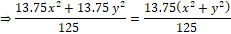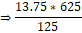Question of The Day14-03-2022

If xcosθ + ysinθ = 24 and xsinθ – ycosθ = 7, then find the value of (13.75x2 + 13.75y2)/125.

Correct Answer : d ) 68.75

Explanation :

According to the question

xcosθ + ysinθ = 24 …..(1)

xsinθ – ycosθ = 7……..(2)

we know that

If xcosθ + ysinθ = a, and

xsinθ – ycosθ = b

then (x2 + y2)(sin2θ + cos2θ) = a2 + b2

comparing eq (1) and (2) with the formula

⇒(x2 + y2)(sin2θ + cos2θ) = 242 + 72

⇒ 576 + 49 = 625

⇒(x2 + y2)(sin2θ + cos2θ) = 625

⇒(x2 + y2) = 625⇒ 68.75

Hence, (d) is the correct answer.0